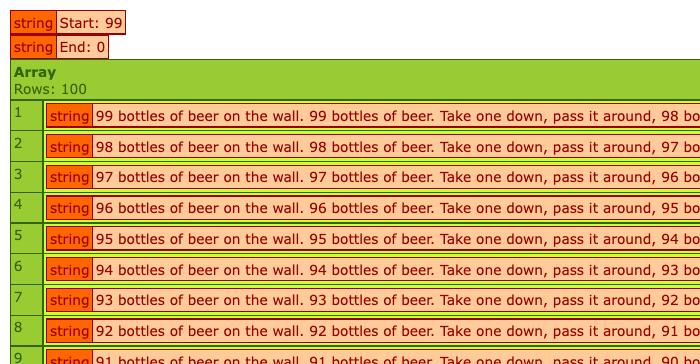# Considering A Numeric Range / Sequence Data Structure In ColdFusion

0
49

I am not sure if I would ever need something like this in a production application, but when I’m toying around with ideas in ColdFusion, it’s not uncommon for me to want to iterate over a sequence of numbers. I know that other languages have the concept of a first class “Range” or “Sequence” structure. And, it seems like something that might be of some value in ColdFusion as well. As such, I wanted to try implementing a numeric range / sequence data structure in Lucee CFML.

The concept of a Range is fairly straightforward as I understand it: there’s an upper-bound and a lower-bound value; and then, there’s functionality for iterating over the values in that static range. For example, you might want to call `.each()` or `.map()` on a range to operator on each value within the range.

I think the easiest way to implement this would be store the upper/lower bound values. And then, as needed, generate an internal Array which would allow us to call the native `.each()` and `.map()` member methods. This would, in turn, bake-in all the native parallel iteration capabilities on top of our numeric range.

Here’s my simple implementation which allows for both ascending and descending ranges:

``````component
accessors = true
output = false
hint = "I provide an iterable sequential numeric range."
{

// Define properties for GETTERS.
property name="endValue" setter=false;
property name="startValue" setter=false;

/**
* I initialize the range with the given outliers (inclusive).
*/
public void function init(
required numeric startValue,
required numeric endValue
) {

variables.startValue = fix( arguments.startValue );
variables.endValue = fix( arguments.endValue );

}

// ---
// PUBLIC METHODS.
// ---

/**
* I iterate over the values in the range using the given operator.
*/
public any function each(
required function operator,
numeric step = 1,
boolean parallel = false,
) {

// NOTE: While it may be more "expensive" to convert to an array first, it makes
// the code significantly easier to write. And, it allows us to leverage the
// native array methods, including the parallelization of the operator.
toArray( step ).each( operator, parallel, maxThreads );

return( this );

}

/**
* I map the values in the range onto an array using the given operator.
*/
public array function map(
required function operator,
numeric step = 1,
boolean parallel = false,
) {

// NOTE: While it may be more "expensive" to convert to an array first, it makes
// the code significantly easier to write. And, it allows us to leverage the
// native array methods, including the parallelization of the operator.
return( toArray( step ).map( operator, parallel, maxThreads ) );

}

/**
* I convert the range into an array using the given step increment.
*/
public array function toArray( numeric step = 1 ) {

var values = [];

step = abs( fix( step ) );

// Range is increasing.
if ( startValue <= endValue ) {

for ( var value = startValue ; value <= endValue ; value += step ) {

values.append( value );

}

// Rance is decreasing.
} else {

for ( var value = startValue ; value >= endValue ; value -= step) {

values.append( value );

}

}

return( values );

}

}
``````

As you can see, there’s really nothing to it. The only method of any consequence is the `.toArray(step)` method. This is what generates the intermediary Array object on which we get to call the native array methods. The other methods – `.each()` and `.map()` – then become little more than proxies to the underlying `.toArray()` method.

In testing / experimentation code, we could then use this to generate numeric ranges for whatever fun purposes we need. For example, we could easily generate the lyrics to the 99 Bottles of Beer children’s song:

``````<cfscript>

range = new Range( 99, 0 );

// Generate the lyrics to the "99 Bottles of Beer" song.
lyrics = range.map(
( value ) => {

if ( ! value ) {

return( "Go home!" );

}

return(
"#value# bottles of beer on the wall. " &
"#value# bottles of beer. " &
"Take one down, pass it around, #(value - 1 )# bottles of beer on the wall."
);

}
);

dump( "Start: " & range.getStartValue() );
dump( "End: " & range.getEndValue() );
dump( lyrics );

</cfscript>
``````

As you can see, we’re creating a descending numeric range from 99 to 0 (inclusive) and then using the `.map()` function to map the range values onto individual lyrics. And, when we run this Lucee CFML code, we get the following output:Again, I am not sure that I would ever need something like this in a production ColdFusion app. Though, maybe I could see it being helpful when generating grid-like interfaces, like a Calendar. But, I would definitely make use of this in a lot of testing / experimentation code.